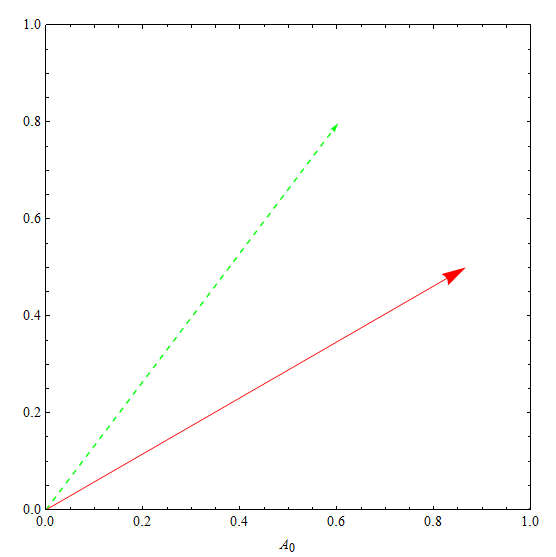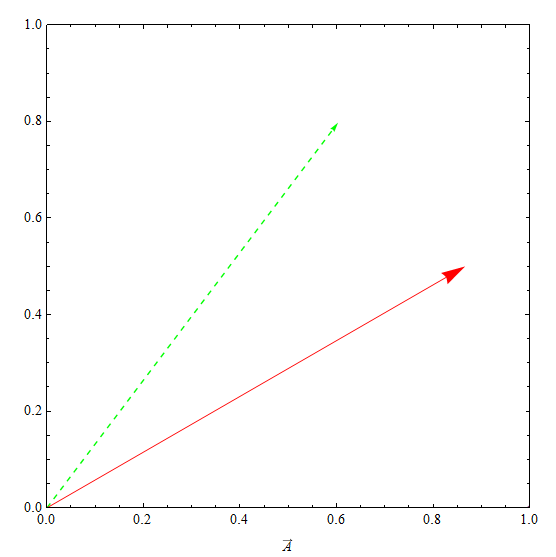$\mathbf{e}^t$是神马含义？$\mathbf{e}^{\text{i} t}$呢?？

“你就先这么理解吧，你取\pi的有限位，可以得到按照上面那种理解方式的一个数，然后你取无限位，就得到这个数了。取无限位的过程你上了大学就知道了，反正就是一个奇奇怪怪的数，呵呵”

“………………….”

“啊，伟大的牛顿，$\text{e}^{t}$是个啥啊？”

“用我发明的微积分，把它展开，它就是”

“额，你这是个啥？”

“用我发明的微积分，他就是$\text{e}^{t} = 1 + t + \frac{t^2}{2!}+ \frac{t^3}{3!}+ \frac{t^4}{4!}+\frac{t^5}{5!}$ …..”

“能说的更明白一些么？”

“用我发明的微积分…..”

$\text{e}$展开后，我们得到了新的理解：“$e^t$这个东西不用再绞尽脑汁怎么去自乘N次开N次方这样去操作e了，我们只需要按照展开式那样，去操作t就行了，然后把这….好吧，无穷多项…..操作加在一起就行了”

## $\text{e}^t$的可视化## $\text{e}^{\text{i}t}$的可视化## 旋转矩阵的可视化

$B\mathbf{A}$的意义是把$\mathbf{A}$的长度变为原来的$\omega$倍，并逆时针旋转$\frac{\pi}{2}$（这和上一个例子一模一样啊！）$\text{e}^B$的意义好直观啊，但是他和我们的旋转矩阵有什么关系呢？

《Visual Complex Analysis》 by Tristan Needham

《Linear Algebra Done Right》by Sheldon Axler

《Linear Algebra and Its Applications(Third Edition)》 by David C. Lay